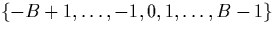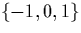Next: Other Possible Representations Up: Approaches to Exact Real Previous: Representation Problems

## Two Suitable Digit Representations

The two digit representations for exact real arithmetic we will use in this work are introduced here. These representations are defined fully in chapter 3. These are suitable representations which allow us to define algorithms to compute basic arithmetic operations, and also higher level transcendental functions.

The representations used require a high degree of redundancy, and in general we cannot compute equality or relational operators on them (although infinite sequences of digits using the standard representations already rejected are slightly redundant, and relational operators are not computable on them either).

The first representation uses one of the most intuitive ways to introduce further redundancy into the representation, which is by allowing negative digits. One way of doing this with the integer base B would be to allow the digitsrather than just the postive ones. The signed binary representation, which is one of those used here, uses digitsThe second representation used consists of a sequence of dyadic rationals, each of which is in the interval [-1,1] and is treated as `pseudo-digit'. Notice that unlike in the first representation, however, there are an infinite number of these `pseudo-digits'.

One of the motivations for these choices of representation is that they are the ones required to implement Alex Simpson's functional operations , which we want to implement and analyse. They also have the advantage that they are relatively easy to represent and understand because of their similarity to more usual unsigned digit representations. Many authors mention these approaches, especially the signed digit one, but as far as we know none develop the full set of arithmetic operations and transcendental functions for them.Next: Other Possible Representations Up: Approaches to Exact Real Previous: Representation Problems
Martin Escardo
5/11/2000